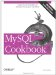# Working with Per-Group and Overall Summary Values Simultaneously

7.17.1 Problem

You want to produce a report that requires different levels of summary detail. Or you want to compare per-group summary values to an overall summary value.

7.17.2 Solution

Use two queries that retrieve different levels of summary information. Or use a programming language to do some of the work so that you can use a single query.

7.17.3 Discussion

Sometimes a report involves different levels of summary information. For example, the following report displays the total number of miles per driver from the driver_log table, along with each driver's miles as a percentage of the total miles in the entire table:

```+-------+--------------+------------------------+
| name | miles/driver | percent of total miles |
+-------+--------------+------------------------+
| Ben | 362 | 16.712834718375 |
| Henry | 911 | 42.059095106187 |
| Suzi | 893 | 41.228070175439 |
+-------+--------------+------------------------+```

The percentages represent the ratio of each driver's miles to the total miles for all drivers. To perform the percentage calculation, you need a per-group summary to get each driver's miles and also an overall summary to get the total miles. Generating the report in SQL involves a couple of queries, because you can't calculate a per-group summary and an overall summary in a single query. First, run a query to get the overall mileage total:

 Well... that's not strictly true. With a subselect, you could generate the summary with a single query. But MySQL won't have subselects until Version 4.1.

```mysql> SELECT @total := SUM(miles) AS 'total miles' FROM driver_log;
+-------------+
| total miles |
+-------------+
| 2166 |
+-------------+```

Then calculate the per-group values and use the overall total to compute the percentages:

```mysql> SELECT name,
-> SUM(miles) AS 'miles/driver',
-> (SUM(miles)*100)/@total AS 'percent of total miles'
-> FROM driver_log GROUP BY name;
+-------+--------------+------------------------+
| name | miles/driver | percent of total miles |
+-------+--------------+------------------------+
| Ben | 362 | 16.712834718375 |
| Henry | 911 | 42.059095106187 |
| Suzi | 893 | 41.228070175439 |
+-------+--------------+------------------------+```

A different form of multiple-query solution that doesn't involve a variable is to retrieve the overall summary into another table, then join that with the original table:

```mysql> CREATE TEMPORARY TABLE t
-> SELECT SUM(miles) AS total FROM driver_log;
mysql> SELECT driver_log.name,
-> SUM(driver_log.miles) AS 'miles/driver',
-> (SUM(driver_log.miles)*100)/t.total AS 'percent of total miles'
-> FROM driver_log, t GROUP BY driver_log.name;
+-------+--------------+------------------------+
| name | miles/driver | percent of total miles |
+-------+--------------+------------------------+
| Ben | 362 | 16.71 |
| Henry | 911 | 42.06 |
| Suzi | 893 | 41.23 |
+-------+--------------+------------------------+```

If you're generating the report from within a program, you can do some of the summary math using your programming language and eliminate one of the queries. Here's an example in Python:

```# issue query to calculate per-driver totals
cursor = conn.cursor ( )
cursor.execute ("SELECT name, SUM(miles) FROM driver_log GROUP BY name")
rows = cursor.fetchall ( )
cursor.close ( )

# iterate once through result to calculate overall total miles
total = 0
for (name, miles) in rows:
total = total + miles

# iterate again to print report
print "name miles/driver percent of total miles"
for (name, miles) in rows:
print "%-8s %5d %f"
% (name, miles, (100*miles)/total)```

Another type of problem that uses different levels of summary information occurs when you want to compare per-group summary values with the corresponding overall summary value. Suppose you want to determine which drivers had a lower average miles per day than the group average. Using only SQL, this task can't be performed with a single query, but you can easily do it with two. First, calculate the overall average and save it in a variable:

```mysql> SELECT @overall_avg := AVG(miles) FROM driver_log;
+----------------------------+
| @overall_avg := AVG(miles) |
+----------------------------+
| 216.6000 |
+----------------------------+```

Then compare each driver's average to the saved value using a HAVING clause:

```mysql> SELECT name, AVG(miles) AS driver_avg FROM driver_log
-> GROUP BY name
-> HAVING driver_avg < @overall_avg;
+-------+------------+
| name | driver_avg |
+-------+------------+
| Ben | 120.6667 |
| Henry | 182.2000 |
+-------+------------+```

Just as when producing a report that uses different levels of summary information, you can solve this problem without using two queries if you're writing a program by using your programming language to do some of the work:

1. Issue a query to retrieve the per-group summary information.
2. Iterate through the result set once to calculate the overall summary value.
3. Iterate through the result set again, comparing each per-group summary value to the overall value and displaying only those records for which the comparison succeeds.MySQL Cookbook
ISBN: 059652708X
EAN: 2147483647
Year: 2005
Pages: 412
Authors: Paul DuBois